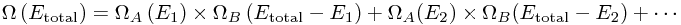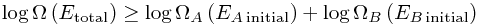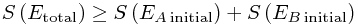So, entropy. Frequently described, in something of a self-fulfilling prophecy, as one of the most confusing concepts in mathematical physics. Let's pretend it's not.

The basic principle underlying statistical physics is called the (rather unimaginative) fundamental assumption of statistical mechanics; it is closely related to something called the ergodic hypothesis. Without concerning ourselves with the mathematical details, it goes something like the following:

### The Fundamental Assumption of Statistical Mechanics

Suppose you have a big, thick-walled box - 1 metre a side - which is filled with a gas. We'll assume the molecules making up the gas are little balls bouncing off each other, which isn't a bad model for a lot of gases. (They'll be shooting around at hundreds of metres per second if we're talking about air at room temperature.) Let's also imagine dividing the space inside up into a million 1cm cubes, and checking how many particles are inside, say once every second.

Now without any particular reason to expect otherwise, it seems natural to expect that - on average - there are the same number of particles in every little cube. And if we divided each little cube into another million even smaller cubes, we'd still expect the same; and so on. Of course, eventually the boxes are so small that most of the time there aren't any molecules in them. At this point, it's more natural to start thinking about probabilities - how likely is it there are some particles in this box?

So let's ask a different sort of question: start by labelling all the particles, P1, P2, P3, ... - and let's label all the boxes, B1, B2, ... - and now we can describe the system (forgetting the velocities of the particles for a moment) by giving a list of where all the particles are, P1 in B54, P2 in B324235, P3 in B42, ... So what's the chance this is what we find when we look at the box? Pretty small! But it's no more particularly unlikely than finding P1 in B2354, P2 in B523423, ...

The content of the fundamental assumption of statistical mechanics is straightforward here: the two possible configurations - the magic word is microstates - have exactly the same probability of being seen. (This applies, though, only to isolated systems which are inequilibrium - so if we've sealed the box and left it alone for a very long time.)

The ergodic hypothesis is a very closely related statement - it basically says that if one waits for a long time, then one observes all possible states in equal numbers.

Aside: One can be much more precise (or alternatively, fussy) in how one states the ergodic hypothesis. A perhaps better way of stating it mathematically involves saying that, over long enough periods of time, the time-average of any quantity Q is the same as the average of Q over all possible configurations. This highlights a particular curiosity of the ergodic hypothesis: there are an absolutely astronomical number of distinguishable configurations (if we look closely enough), and exploring them all would take considerably longer than the age of the universe. This means that getting the right 'average' for a very specific observable Q like "the number of particles in this molecule-sized region of space" would take a ridiculous length of time. But on the other hand, something like "the total pressure exerted on the near wall of the whole box" not only has fluctuations so tiny you would basically never notice it (which makes sense, because you're averaging over a huge number of particles), but agrees to incredible precision with the average over the whole configuration space - even though the system only has time to explore a minuscule portion of the whole space! This is telling us that the system has a lot of symmetry (for example, we can swap pairs of particles - thinking classically - and this new region of space looks exactly the same as the old one, just spun around a bit), to a truly remarkable extent.

### States

What is this probability of being seen? Well, it's 1/Ω, where Ω (capital omega) is the total number of possible configurations which were allowed in the first place. And this extends to any other description of a "configuration", however much or little we describe about the system. For example, Ω is different if the box is bigger, or if there are more particles - thus Ω is a function that changes when we change the volume V or the number of particles N.

But this is an interesting point - how much detail do we give when we describe the system? In our normal lives, we're not interested in the locations of specific particles and so on (which is just as well since it would be impossible to actually find this out), so the only things we know are bulk properties of the system. In fact, to all intents and purposes, we can usually describe the system completely by only a few variables, or functions of state. These thermodynamic variables include things like energy E, particle number N, temperature T, volume V and pressure P - and they aren't all independent! If you change the volume of a gas, you can't keep everything else fixed; usually, it has to change the pressure it exerts, or its temperature.

That is, pretty much everything we can hope to know about a gas in a box can be described by listing - say - N, V and T. This is much neater than worrying about lots of individual particles flying about; all the information we care about there gets wrapped up into the function Ω(N, V, T), which tells us how horrifically complicated the system is. This is all well and good, and you might think "Who cares about this Ω?"

### The Second Law of Thermodynamics

All the interesting stuff happens when we expand our budget to buy a second box. Let's just suppose that the two boxes are allowed to exchange energy E - through a metal wall, say - and forget about the other thermodynamic variables. Okay. Now we have two boxes A and B, and a Ω for each, ΩA(E1) and ΩB(E2). We start with the boxes separate, and then let them come together. The only law we have to worry about is conservation of energy - so we need the total energy Etotal to be the same before and after. That is, the combined system has to have energy EA initial + EB initial.

How many possible microstates are there? To begin with, there were ΩA(EA initial) × ΩB(EB initial). But afterwards, we don't know that they have the same energies. We can let A have any allowed energy Ei, and then B gets Etotal - Ei. So we need to add up all the different ways energy could be divided, as they all contribute some number of microstates:Now somewhere in that sum, we get Ei = EA initial - so the whole sum is at least as big as the original total number of microstates:To put this in words,

There are more ways to arrange everything when we can move energy between the systems.

Hooray! That is pretty much the second law of thermodynamics. The only mathematical trick left is to take logarithms. This is a good idea because log(ab) = log(a) + log(b), so that we getThen we invent a name for this logarithm, and define entropy to be basically exactly this, up to a random constant (Boltzmann's constant) : S = kB log Ω. Thusor: total entropy always increases! Note that the logarithm is an increasing function, so a bigger Ω always means a bigger S and vice versa - this is just a convenient rescaling.

### Most Likely State - The Notion of Temperature

What about if we separate the systems again? Or more simply, we peer in and see just how much energy system A has got. Of course, this is a variable which fluctuates over time as energy sloshes between the systems - although we'll see this doesn't happen very much. Intuitively, we expect the bodies to exchange energy until they reach the same 'temperature', at which point everything is in equilibrium again.

Well, we need to go back to our big sum:Each term tells us what number of the total states have the energy shared out in a particular way - with A getting E1 of the energy - so we know the probability that A has every possible energy! But we can actually do much better by thinking about the size of the Ωs.

These Ωs are BIG.

[more to come...]